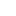# Total expenditure, average expenditure and distribution of household expenditure

1.1 Total expenditure, average expenditure and percentage distribution of expenditure according to different levels of functional breakdown
2.2 Total expenditure, average expenditure and percentage distribution of total expenditure and index on the mean of the average expenditure per person (a.e.p) according to different classification variables
1.Última modificación:
07/28/2020
Dimensiones:
Periodo:
2.1 Total expenditure and average expenditure by size of the municipality of residence
2.Última modificación:
07/28/2020
Dimensiones:
Periodo:
2.2 Total expenditure and average expenditure by population density
3.Última modificación:
07/28/2020
Dimensiones:
Periodo:
2.3 Total expenditure and average expenditure by sex and age of main breadwinner
4.Última modificación:
07/28/2020
Dimensiones:
Periodo:
2.4 Total expenditure and average expenditure by educational level of the main breadwinner.
5.Última modificación:
07/28/2020
Dimensiones:
Periodo:
2.5 Total expenditure and average expenditure by economic activity situation of the main breadwinner.
6.Última modificación:
07/28/2020
Dimensiones:
Periodo:
2.6 Total expenditure and average expenditure by professional situation of the main breadwinner.
7.Última modificación:
07/28/2020
Dimensiones:
Periodo:
2.7 Total expenditure and average expenditure by occupancy CNO-11 (as of 2012), (large groups) of the main breadwinner.
8.Última modificación:
07/28/2020
Dimensiones:
Periodo:
2.8 Total expenditure and average expenditure by Size of household.
9.Última modificación:
07/28/2020
Dimensiones:
Periodo:
2.9 Total expenditure and average expenditure by household type.
10.Última modificación:
07/28/2020
Dimensiones:
Periodo:
2.10 Total expenditure and average expenditure by main source of household income.
11.Última modificación:
07/28/2020
Dimensiones:
Periodo:
2.11 Total expenditure and average expenditure by level of regular net monthly household income.
12.Última modificación:
07/28/2020
Dimensiones:
Periodo:
2.12 Total expenditure and average expenditure by nationality (Spanish or foreign) of the main breadwinner.
13.Última modificación:
07/28/2020
Dimensiones:
Periodo:
2.13 Total expenditure and average expenditure by country of birth of the main breadwinner (as of 2011).
14.Última modificación:
07/28/2020
Dimensiones:
Periodo:
2.14 Total expenditure and average expenditure by country of birth of the main breadwinner's parents (as of 2011).
3.3 Average expenditure per household, average expenditure per person and percentage distribution of total expenditure (2 digits) according to different classification variables
4.4 Percentage distribution of expenditure by expenditure subgroup (3 digits), according to different classification variables
5.5 Average expenditure per household, average expenditure per person and percentage of households with expenditure in certain codes (list of codes up to 5 digits ECOICOP/EPF)
6.6 Means household expense, mean expense per person and distribution of the expense (vertical and horizontal percentages) by expense groups and by quintile of the expense
7.7 Expenditure on Internet purchases